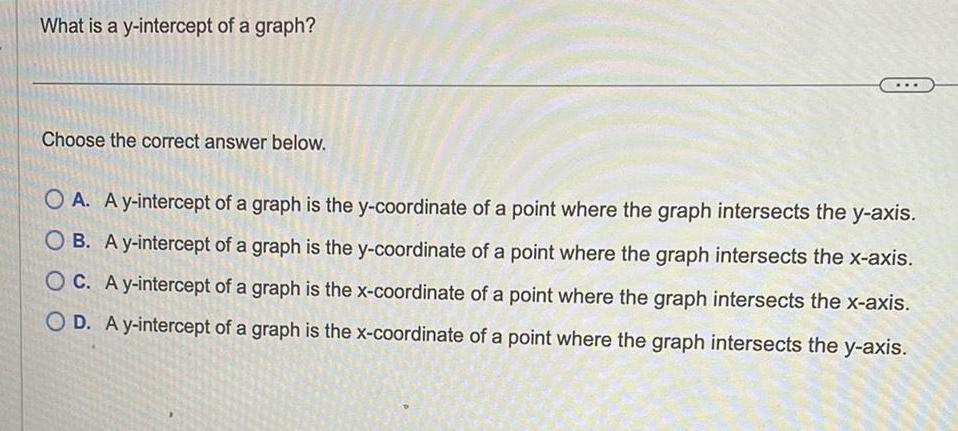Question:

# What is a y intercept of a graph Choose the correct answer

Last updated: 9/18/2023What is a y intercept of a graph Choose the correct answer below OA A y intercept of a graph is the y coordinate of a point where the graph intersects the y axis OB A y intercept of a graph is the y coordinate of a point where the graph intersects the x axis OC A y intercept of a graph is the x coordinate of a point where the graph intersects the x axis OD A y intercept of a graph is the x coordinate of a point where the graph intersects the y axis